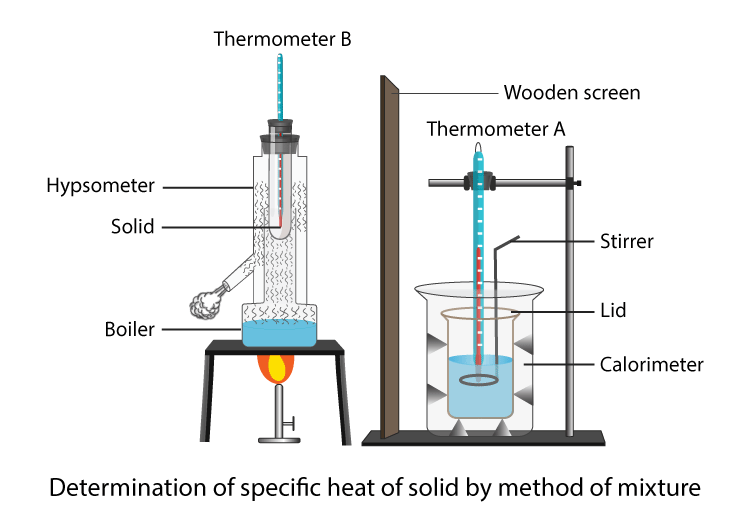# Explain The Procedure Of Finding Specific Heat Of Solid Experimentally?

Specific heat is the quantity of heat required to raise the temperature of any substance by 1 degree Celsius.

Specific Heat formula is

C= $$\frac{\Delta Q}{m \Delta T}$$

Where,

m is the mass

Δ Q is the heat gained or lost

Δ T is the temperature differenceThe procedure to determine the specific heat capacity of a solid:

• A calorimeter measures the specific heat capacity of a solid.
• Arrange all the apparatus as shown in the above diagram.
• Place the thermometers A and B in a beaker containing water and make a note of their reading.
• Allow thermometer A to be a standard to find the correction that is to be applied to thermometer B
• Place the thermometer B into the copper tube of a hypsometer containing the powder of the given solid.
• Now place the hypsometer on the burner by adding a sufficient amount of water.
• Record the weight of calorimeter along with stirrer and lid over it
• Make a note of the temperature. Add water when the temperature is between 5℃ to 8℃ and up to the half-length of the calorimeter
• Record the weight of calorimeter along with stirrer and lid over it
• Heat the hypsometer till the temperature of the solid is steady.
• Note the temperature of water in calorimetry.
• Add solid powder from the hypsometer into the calorimeter
• Record the final temperature of the mixture.
• Remove the thermometer from the calorimeter and weigh the calorimeter with the contents and lid.
• Now, as per the obtained values, calculate the specific heat capacity of a solid.

Explore more such questions and answers at BYJU’S.